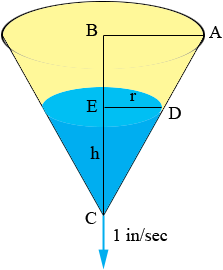SEARCH HOMEMath Central Quandaries & QueriesQuestion from Rachael, a student: Hello, I am a 10th grader in AP Calc, and can not figure out this question: Water is running out of a conical funnel at the rate of 1 inch^3/sec. If the radius of the base of the funnel is 4 in. and the altitude is 8 in., find the rate at which the water level is dropping when it is 2 in. from the top.Hi Rachael,Let the radius and height of the water in the cone at time t seconds be r and h inches. The volume of the cone of water is then V = 1/3 π r2 h cubic inches. V, h and r are all functions of time t. Triangles ABC and DEC are similar and |BC| is twice |AB| so h = 2r. You are told that dV/dt = -1 in3/sec and you want to find dh/dt at a particular time, the tie when |BE| = 2 inches. Use the fact that h = 2r (ie r = h/2) to express V as a function of h alone. Differentiate this expression implicitly with respect to t. This will result in an expression containing dV/dt, h and dh/dt. dV/dt and h you know at the time in question so you can solve for dh/dt.

HarleyMath Central is supported by the University of Regina and The Pacific Institute for the Mathematical Sciences.